# NCERT Solution Class 10 Maths Chapter 10 – Circles

## Exercise: 10.1 Class 10 Maths NCERT Solution (Page No: 209)

1. How many tangents can a circle have?

There can be infinite tangents to a circle. A circle is made up of infinite points which are at an equal distance from a point. Since there are infinite points on the circumference of a circle, infinite tangents can be drawn from them.

2. Fill in the blanks:

(i) A tangent to a circle intersects it in …………… point(s).

(ii) A line intersecting a circle in two points is called a ………….

(iii) A circle can have …………… parallel tangents at the most.

(iv) The common point of a tangent to a circle and the circle is called …………

(i) A tangent to a circle intersects it in one point(s).

(ii) A line intersecting a circle in two points is called a secant.

(iii) A circle can have two parallel tangents at the most.

(iv) The common point of a tangent to a circle and the circle is called the point of contact.

3. A tangent PQ at a point P of a circle of radius 5 cm meets a line through the centre O at

a point Q so that OQ = 12 cm. Length PQ is :

(A) 12 cm

(B) 13 cm

(C) 8.5 cm

(D) √119 cm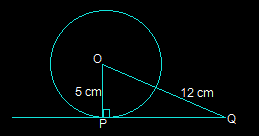In the above figure, the line that is drawn from the centre of the given circle to the tangent PQ is perpendicular to PQ.

And so, OP ⊥ PQ

Using Pythagoras theorem in triangle ΔOPQ we get,

OQ2 = OP2+PQ2

(12)= 52+PQ2

PQ2 = 144-25

PQ2 = 119

PQ = √119 cm

So, option D i.e. √119 cm is the length of PQ.

4. Draw a circle and two lines parallel to a given line such that one is a tangent and the

other, a secant to the circle.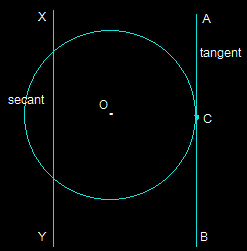In the above figure, XY and AB are two the parallel lines. The line segment AB is the tangent at point C while the line segment XY is the secant.

## Exercise: 10.2 Class 10 Maths NCERT Solution (Page NO: 213)

In Q.1 to 3, choose the correct option and give justification.

1. From a point Q, the length of the tangent to a circle is 24 cm and the distance of Q from the centre is 25 cm. The radius of the circle is

(A) 7 cm

(B) 12 cm

(C) 15 cm

(D) 24.5 cm

First, draw a perpendicular from the center O of the triangle to a point P on the circle which is touching the tangent. This line will be perpendicular to the tangent of the circle.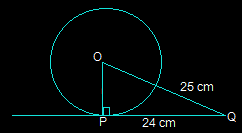So, OP is perpendicular to PQ i.e. OP ⊥ PQ

From the above figure, it is also seen that △OPQ is a right angled triangle.

It is given that

OQ = 25 cm and PQ = 24 cm

By using Pythagoras theorem in △OPQ,

OQ2 = OP2 +PQ2

(25)= OP2+(24)2

OP2 = 625-576

OP2 = 49

OP = 7 cm

So, option A i.e. 7 cm is the radius of the given circle.

2. In Fig. 10.11, if TP and TQ are the two tangents to a circle with centre O so that ∠POQ = 110°, then ∠PTQ is equal to

(A) 60°

(B) 70°

(C) 80°

(D) 90°

From the question, it is clear that OP is the radius of the circle to the tangent PT and OQ is the radius to the tangents TQ.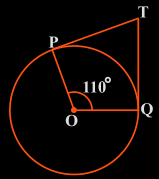So, OP ⊥ PT and TQ ⊥ OQ

∴∠OPT = ∠OQT = 90°

Now, in the quadrilateral POQT, we know that the sum of the interior angles is 360°

So, ∠PTQ+∠POQ+∠OPT+∠OQT = 360°

Now, by putting the respective values we get,

∠PTQ +90°+110°+90° = 360°

∠PTQ = 70°

So, ∠PTQ is 70° which is option B.

3. If tangents PA and PB from a point P to a circle with centre O are inclined to each other at angle of 80°, then ∠ POA is equal to

(A) 50°

(B) 60°

(C) 70°

(D) 80°

First, draw the diagram according to the given statement.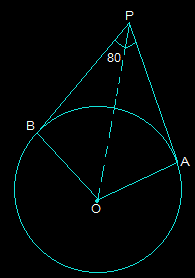Now, in the above diagram, OA is the radius to tangent PA and OB is the radius to tangents PB.

So, OA is perpendicular to PA and OB is perpendicular to PB i.e. OA ⊥ PA and OB ⊥ PB

So, ∠OBP = ∠OAP = 90°

The sum of all the interior angles will be 360°

So, ∠AOB+∠OAP+∠OBP+∠APB = 360°

Putting their values, we get,

∠AOB + 260° = 360°

∠AOB = 100°

Now, consider the triangles △OPB and △OPA. Here,

AP = BP (Since the tangents from a point are always equal)

OA = OB (Which are the radii of the circle)

OP = OP (It is the common side)

Now, we can say that triangles OPB and OPA are similar using SSS congruency.

∴△OPB ≅ △OPA

So, ∠POB = ∠POA

∠AOB = ∠POA+∠POB

2 (∠POA) = ∠AOB

By putting the respective values, we get,

=>∠POA = 100°/2 = 50°

As angle ∠POA is 50° option A is the correct option.

4. Prove that the tangents drawn at the ends of a diameter of a circle are parallel.

First, draw a circle and connect two points A and B such that AB becomes the diameter of the circle. Now, draw two tangents PQ and RS at points A and B respectively.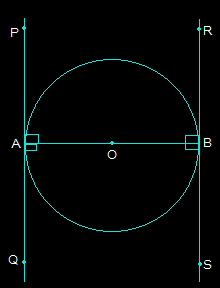Now, both radii i.e. AO and OP are perpendicular to the tangents.

So, OB is perpendicular to RS and OA perpendicular to PQ

So, ∠OAP = ∠OAQ = ∠OBR = ∠OBS = 90°

From the above figure, angles OBR and OAQ are alternate interior angles.

Also, ∠OBR = ∠OAQ and ∠OBS = ∠OAP (Since they are also alternate interior angles)

So, it can be said that line PQ and the line RS will be parallel to each other. (Hence Proved).

5. Prove that the perpendicular at the point of contact to the tangent to a circle passes through the center.

Solution:

First, draw a circle with center O and draw a tangent AB which touches the radius of the circle at point P.

To Proof: PQ passes through point O.

Now, let us consider that PQ doesn’t pass through point O. Also, draw a CD parallel to AB through O. Here, CD is a straight line and AB is the tangent. Refer the diagram now.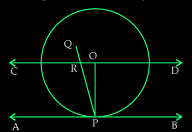From the above diagram, PQ intersects CD and AB at R and P respectively.

AS, CD ∥ AB,

Here, the line segment PQ is the line of intersection.

Now angles ORP and RPA are equal as they are alternate interior angles

So, ∠ORP = ∠RPA

And,

∠RPA = 90° (Since, PQ is perpendicular to AB)

∠ORP = 90°

Now, ∠ROP+∠OPA = 180° (Since they are co-interior angles)

∠ROP+90° = 180°

∠ROP = 90°

Now, it is seen that the △ORP has two right angles which are ∠ORP and ∠ROP. Since this condition is impossible, it can be said the supposition we took is wrong.

6. The length of a tangent from a point A at distance 5 cm from the centre of the circle is 4 cm. Find the radius of the circle.

Draw the diagram as shown below.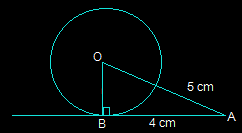Here, AB is the tangent that is drawn on the circle from a point A.

So, the radius OB will be perpendicular to AB i.e. OB ⊥ AB

We know, OA = 5cm and AB = 4 cm

Now, In △ABO,

OA2 =AB2+BO(Using Pythagoras theorem)

5= 42+BO2

BO2 = 25-16

BO2 = 9

BO = 3

So, the radius of the given circle i.e. BO is 3 cm.

7. Two concentric circles are of radii 5 cm and 3 cm. Find the length of the chord of the larger circle which touches the smaller circle.

Draw two concentric circles with the center O. Now, draw a chord AB in the larger circle which touches the smaller circle at a point P as shown in the figure below.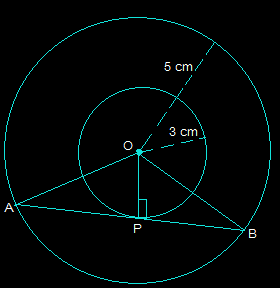From the above diagram, AB is tangent to the smaller circle to point P.

∴ OP ⊥ AB

Using Pythagoras theorem in triangle OPA,

OA2= AP2+OP2

52 = AP2+32

AP2 = 25-9

AP = 4

Now, as OP ⊥ AB,

Since the perpendicular from the center of the circle bisects the chord, AP will be equal to PB

So, AB = 2AP = 2×4 = 8 cm

So, the length of the chord of the larger circle is 8 cm.

8. A quadrilateral ABCD is drawn to circumscribe a circle (see Fig. 10.12). Prove that AB + CD = AD + BC

The figure given is: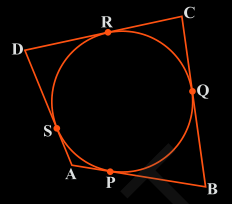From this figure we can conclude a few points which are:

(i) DR = DS

(ii) BP = BQ

(iii) AP = AS

(iv) CR = CQ

Since they are tangents on the circle from points D, B, A, and C respectively.

Now, adding the LHS and RHS of the above equations we get,

DR+BP+AP+CR = DS+BQ+AS+CQ

By rearranging them we get,

(DR+CR) + (BP+AP) = (CQ+BQ) + (DS+AS)

By simplifying,

9. In Fig. 10.13, XY and X′Y′ are two parallel tangents to a circle with centre O and another tangent AB with point of contact C intersecting XY at A and X′Y′ at B. Prove that ∠ AOB = 90°.

From the figure given in the textbook, join OC. Now, the diagram will be as-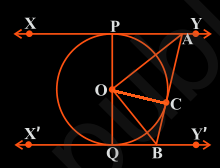Now the triangles △OPA and △OCA are similar using SSS congruency as:

(i) OP = OC They are the radii of the same circle

(ii) AO = AO It is the common side

(iii) AP = AC These are the tangents from point A

So, △OPA ≅ △OCA

Similarly,

△OQB ≅ △OCB

So,

∠POA = ∠COA … (Equation i)

And, ∠QOB = ∠COB … (Equation ii)

Since the line POQ is a straight line, it can be considered as a diameter of the circle.

So, ∠POA +∠COA +∠COB +∠QOB = 180°

Now, from equations (i) and equation (ii) we get,

2∠COA+2∠COB = 180°

∠COA+∠COB = 90°

∴∠AOB = 90°

10. Prove that the angle between the two tangents drawn from an external point to a circle is supplementary to the angle subtended by the line-segment joining the points of contact at the center.

First, draw a circle with centre O. Choose an external point P and draw two tangents PA and PB at point A and point B respectively. Now, join A and B to make AB in a way that it subtends ∠AOB at the center of the circle. The diagram is as follows: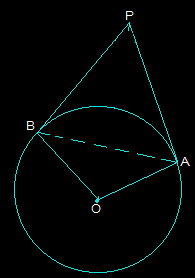From the above diagram, it is seen that the line segments OA and PA are perpendicular.

So, ∠OAP = 90°

In a similar way, the line segments OB ⊥ PB and so, ∠OBP = 90°

∴∠APB+∠OAP +∠PBO +∠BOA = 360° (since the sum of all interior angles will be 360°)

By putting the values we get,

∠APB + 180° + ∠BOA = 360°

So, ∠APB + ∠BOA = 180° (Hence proved).

11. Prove that the parallelogram circumscribing a circle is a rhombus.

Consider a parallelogram ABCD which is circumscribing a circle with a center O. Now, since ABCD is a parallelogram, AB = CD and BC = AD.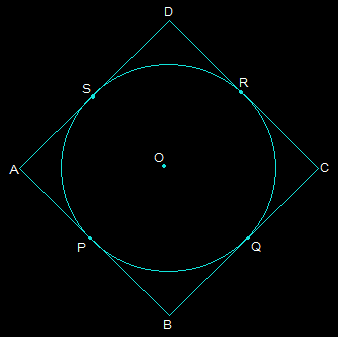From the above figure, it is seen that,

(i) DR = DS

(ii) BP = BQ

(iii) CR = CQ

(iv) AP = AS

These are the tangents to the circle at D, B, C, and A respectively.

DR+BP+CR+AP = DS+BQ+CQ+AS

By rearranging them we get,

(BP+AP)+(DR+CR) = (CQ+BQ)+(DS+AS)

Again by rearranging them we get,

Now, since AB = CD and BC = AD, the above equation becomes

2AB = 2BC

∴ AB = BC

Since AB = BC = CD = DA, it can be said that ABCD is a rhombus.

12. A triangle ABC is drawn to circumscribe a circle of radius 4 cm such that the segments BD and DC into which BC is divided by the point of contact D are of lengths 8 cm and 6 cm respectively (see Fig. 10.14). Find the sides AB and AC.

The figure given is as follows: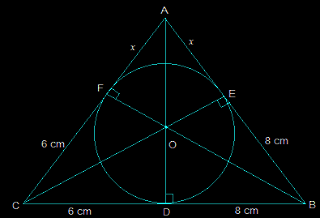Consider the triangle ABC,

We know that the length of any two tangents which are drawn from the same point to the circle is equal.

So,

(i) CF = CD = 6 cm

(ii) BE = BD = 8 cm

(iii) AE = AF = x

Now, it can be observed that,

(i) AB = EB+AE = 8+x

(ii) CA = CF+FA = 6+x

(iii) BC = DC+BD = 6+8 = 14

Now the semi perimeter “s” will be calculated as follows

2s = AB+CA+BC

By putting the respective values we get,

2s = 28+2x

s = 14+x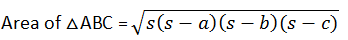By solving this we get,

= √(14+x)48x ……… (i)

Again, the area of △ABC = 2 × area of (△AOF + △COD + △DOB)

= 2×[(½×OF×AF)+(½×CD×OD)+(½×DB×OD)]

= 2×½(4x+24+32) = 56+4…………..(ii)

Now from (i) and (ii) we get,

√(14+x)48= 56+4x

Now, square both the sides,

48x(14+x) = (56+4x)2

48x = [4(14+x)]2/(14+x)

48x = 16(14+x)

48x = 224+16x

32x = 224

x = 7 cm

So, AB = 8+x

i.e. AB = 15 cm

And, CA = x+6 =13 cm.

13. Prove that opposite sides of a quadrilateral circumscribing a circle subtend supplementary angles at the centre of the circle.

First draw a quadrilateral ABCD which will circumscribe a circle with its centre O in a way that it touches the circle at point P, Q, R, and S. Now, after joining the vertices of ABCD we get the following figure: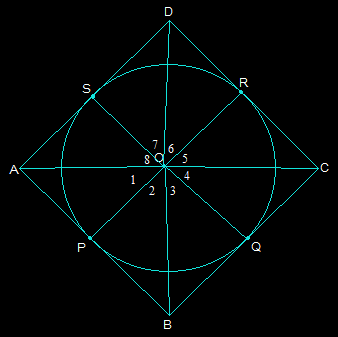Now, consider the triangles OAP and OAS,

AP = AS (They are the tangents from the same point A)

OA = OA (It is the common side)

OP = OS (They are the radii of the circle)

So, by SSS congruency △OAP ≅ △OAS

So, ∠POA = ∠AOS

Which implies that∠1 = ∠8

Similarly, other angles will be,

∠4 = ∠5

∠2 = ∠3

∠6 = ∠7

Now by adding these angles we get,

∠1+∠2+∠3 +∠4 +∠5+∠6+∠7+∠8 = 360°

Now by rearranging,

(∠1+∠8)+(∠2+∠3)+(∠4+∠5)+(∠6+∠7) = 360°

2∠1+2∠2+2∠5+2∠6 = 360°

Taking 2 as common and solving we get,

(∠1+∠2)+(∠5+∠6) = 180°

Thus, ∠AOB+∠COD = 180°

Similarly, it can be proved that ∠BOC+∠DOA = 180°

Therefore, the opposite sides of any quadrilateral which is circumscribing a given circle will subtend supplementary angles at the center of the circle.